# Lithium Battery Charging and Discharging Theory and Design of Battery Capacity Calculation Method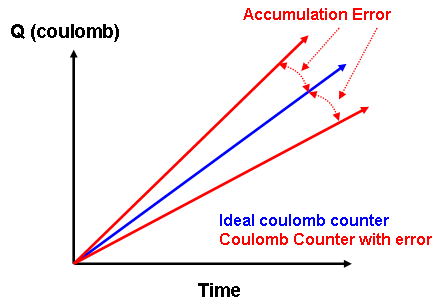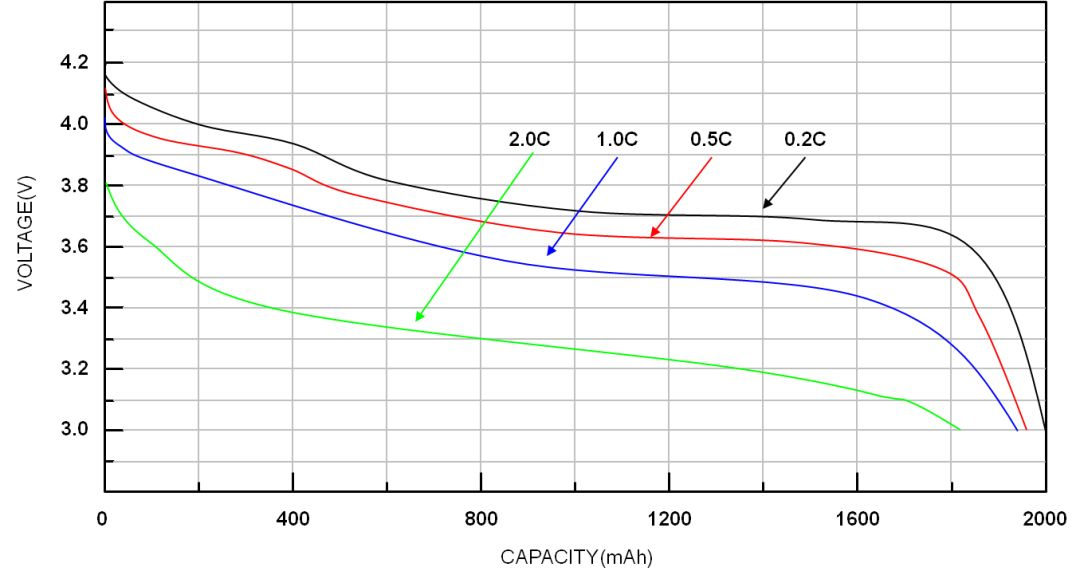1. Introduction to lithium-ion batteries

1.1 State-Of-Charge;SOC

The state of charge can be defined as the available energy state of a battery, usually expressed as a percentage. Since the available energy can vary due to charging/discharging current, temperature, and aging phenomena, the definition of state of charge is also divided into two types: Absolute State of Charge (ASOC) and Relative State of Charge (RSOC). Typically, the range of RSOC is from 0% to 100%, where 100% represents a fully charged battery and 0% represents a fully discharged battery. ASOC, on the other hand, is a reference value calculated based on the fixed capacity value designed when the battery is manufactured. The ASOC of a brand new fully charged battery is 100%, but an aged battery, even when fully charged, may not reach 100% under different charging/discharging conditions.

The graph below illustrates the relationship between voltage and battery capacity at different discharge rates. As the discharge rate increases, the battery capacity decreases. Additionally, battery capacity also decreases at lower temperatures.Figure 1. The relationship between voltage and capacity at different discharge rates and temperatures.

1.2 Max Charging Voltage

The maximum charging voltage of a battery is determined by its chemical composition and characteristics. For lithium batteries, the charging voltage typically ranges between 4.2V and 4.35V. However, the voltage values may vary depending on the specific cathode and anode materials used in the battery.

1.3 Fully Charged

When the voltage difference between the battery voltage and the maximum charging voltage is less than 100mV, and the charging current is reduced to C/10, the battery can be considered fully charged. The specific conditions for a battery to be considered fully charged may vary depending on its characteristics.

The graph below represents a typical charging characteristic curve of a lithium battery. When the battery voltage reaches the maximum charging voltage and the charging current is reduced to C/10, the battery is considered fully charged.

1.4 Mini Discharging Voltage

The minimum discharge voltage can be defined as the cutoff discharge voltage, which is typically the voltage when the state of charge is 0%. This voltage value is not fixed but changes with factors such as load, temperature, degree of aging, or others.

1.5 Fully Discharge

When the battery voltage is less than or equal to the minimum discharge voltage, it can be referred to as a complete discharge.

1.6 C-Rate

The charge/discharge rate is a representation of the charging or discharging current relative to the battery capacity. For example, if a battery is discharged at 1C, ideally, it would be completely discharged after one hour. Different charge/discharge rates result in different available capacities. Generally, higher charge/discharge rates lead to smaller available capacities.

1.7 The cycle count refers to the number of complete charge and discharge cycles that a battery undergoes and can be estimated based on the actual discharged capacity and the design capacity. Each time the accumulated discharged capacity reaches the design capacity, it counts as one cycle. Typically, after around 500 charge and discharge cycles, the fully charged battery capacity can decrease by approximately 10% to 20%.

1.8 Self-Discharge

The self-discharge of all batteries increases with temperature. Self-discharge is essentially not a manufacturing defect but rather an inherent characteristic of batteries. However, improper handling during the manufacturing process can also contribute to increased self-discharge. Typically, for every 10°C increase in temperature, the self-discharge rate doubles. Lithium-ion batteries have a self-discharge rate of approximately 1-2% per month, while various nickel-based batteries have a self-discharge rate of approximately 10-15% per month.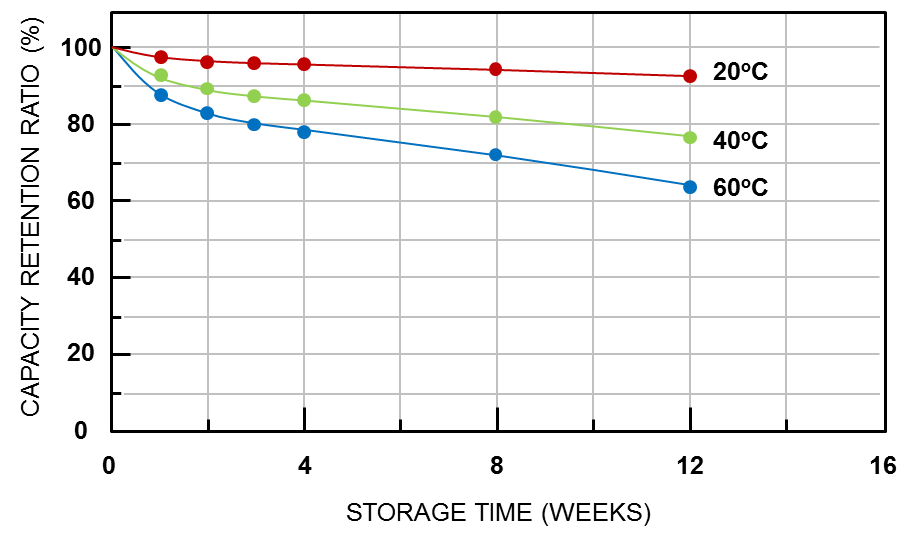Figure 4. Performance of lithium battery self-discharge rate at different temperatures
• 2.1 Introduction to Battery Capacity Gauge
• 3.2.1 Introduction to Battery Fuel Gauge Functionality

Battery management can be seen as part of power management. In battery management, the battery capacity gauge is responsible for estimating the battery capacity. Its basic function is to monitor voltage, charging/discharging current, and battery temperature, and estimate the state of charge (SOC) and the full charge capacity (FCC) of the battery.There are two typical methods for estimating the state of charge (SOC) of a battery: open-circuit voltage (OCV) method and coulomb counting method. Another method is the dynamic voltage algorithm designed by RICHTEK.

2.2 open circuit voltage method

With an open-circuit voltage (OCV) method-based fuel gauge, the implementation process is relatively straightforward. It involves referencing a lookup table that correlates open-circuit voltage with the corresponding state of charge (SOC). The OCV method assumes that the battery has been at rest for approximately 30 minutes, and the measured voltage during this resting period represents the battery terminal voltage.

Different loads, temperatures, and battery aging conditions can result in variations in the battery voltage curve. Therefore, a fixed lookup table for open-circuit voltage cannot fully represent the state of charge (SOC). It is not reliable to rely solely on lookup tables to estimate the SOC. In other words, if the SOC is estimated solely based on lookup tables, there will be a significant margin of error.

Based on the provided figure, it is evident that there is a significant difference in the state of charge (SOC) under different loads during discharge. Therefore, in general, the open-circuit voltage (OCV) method is only suitable for systems with low accuracy requirements for SOC estimation, such as automotive applications using lead-acid batteries or uninterruptible power supplies (UPS).

2.3 coulomb counting method

The operating principle of the coulomb counting method involves connecting a sensing resistor in the charging/discharging path of the battery. An analog-to-digital converter (ADC) measures the voltage across the sensing resistor, which is then converted into the current value flowing into or out of the battery during charging or discharging. A real-time counter (RTC) is used to integrate this current value over time, providing information about the amount of coulombs that have flowed through the battery.

The coulomb counting method allows for accurate calculation of the real-time state of charge (SOC) during charging or discharging processes. By using the charging coulomb counter and discharging coulomb counter, it can calculate the remaining capacity (RM) and the full charge capacity (FCC). The SOC can be derived from the remaining capacity (RM) and full charge capacity (FCC) using the formula SOC = RM / FCC. Additionally, it can also estimate the remaining time, such as time to empty (TTE) and time to full (TTF).

There are two main factors that contribute to the deviation in accuracy of the coulomb counting method. The first factor is the accumulation of offset errors in current sensing and ADC measurements. Although the current measurement error is relatively small with current technology, without proper correction methods, this error can increase over time. The following figure demonstrates that in practical applications, if no correction is applied over time, the accumulated error is unbounded.

To eliminate accumulated errors, there are three potential time points that can be used during normal battery operation: End of Charge (EOC), End of Discharge (EOD), and Relaxation. The EOC condition indicates that the battery is fully charged, and the state of charge (SOC) should be 100%. The EOD condition indicates that the battery is completely discharged, and the SOC should be 0%. It can be defined by an absolute voltage value or can vary with the load. The Relaxation state is reached when the battery is neither charging nor discharging and remains in this state for a significant period of time. If the user intends to use the relaxation state for error correction in the coulomb counting method, it must be combined with an open-circuit voltage table. The following figure demonstrates that under the aforementioned conditions, the state of charge error can be corrected.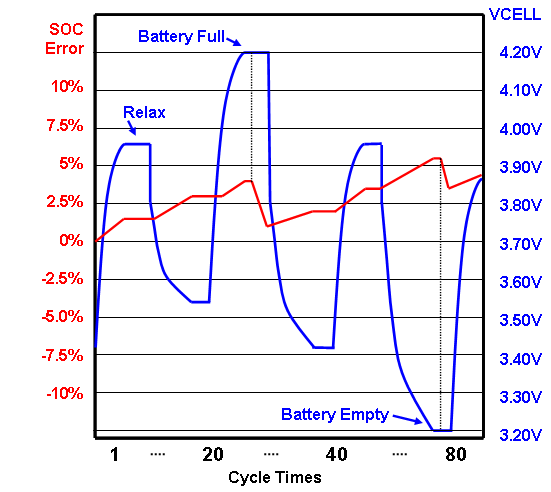Figure 10. Conditions for eliminating the cumulative error of the coulometric method

The second major factor causing deviations in Coulomb counting accuracy is the Full Charge Capacity (FCC) error, which arises from the difference between the battery’s designed capacity and its actual full charge capacity. The FCC can be influenced by factors such as temperature, aging, and load conditions. Therefore, methods for relearning and compensating for the Full Charge Capacity are crucial for Coulomb counting.

The chart below illustrates the trend of state-of-charge errors when the Full Charge Capacity is overestimated or underestimated.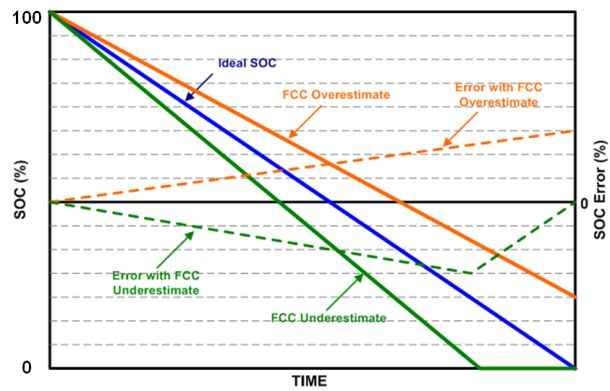Figure 11. The trend of error when the full charge capacity is overestimated and underestimated

2.4 Dynamic Voltage Algorithm Fuel Gauge

The Dynamic Voltage Algorithm is a method for estimating the state of charge (SOC) of a lithium battery solely based on its voltage. It calculates the incremental or decremental change in the SOC by considering the difference between the battery voltage and its open-circuit voltage. The dynamic voltage information effectively simulates the behavior of the lithium battery and determines the SOC percentage, but it does not estimate the battery capacity value in milliampere-hours (mAh).

The Dynamic Voltage Algorithm calculates the incremental or decremental change in the state of charge (SOC) by utilizing the dynamic differences between the battery voltage and the open-circuit voltage. It employs an iterative algorithm to estimate the SOC based on these voltage differentials. Compared to the Coulomb counting method, the Dynamic Voltage Algorithm does not accumulate errors over time and current.

The Coulomb counting method for SOC estimation is prone to errors due to current sensing inaccuracies and battery self-discharge, leading to inaccurate SOC estimation. Even with very small current sensing errors, the Coulomb counter will continue to accumulate errors, which can only be eliminated when the battery is fully charged or discharged.

On the other hand, the Dynamic Voltage Algorithm estimates the battery’s SOC solely based on voltage information, without relying on current information, thus avoiding error accumulation. To improve the accuracy of SOC estimation, the Dynamic Voltage Algorithm requires the optimization of algorithm parameters based on the actual battery voltage curves obtained from real devices under fully charged and fully discharged conditions.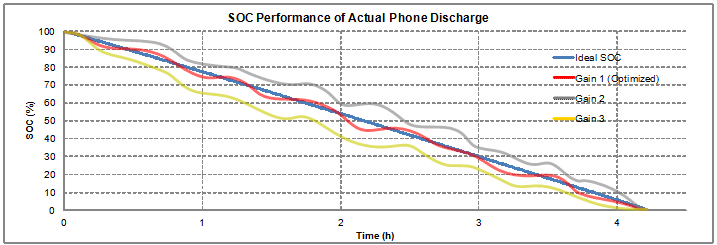Figure 12. Performance of Dynamic Voltage Algorithm Fuel Gauge and Gain Optimization

The following table shows the performance of the Dynamic Voltage Algorithm in different discharge rate conditions, indicating its good accuracy in estimating the state of charge (SOC). According to the table, regardless of the discharge conditions such as C/2, C/4, C/7, and C/10, the overall SOC error of this method is less than 3%.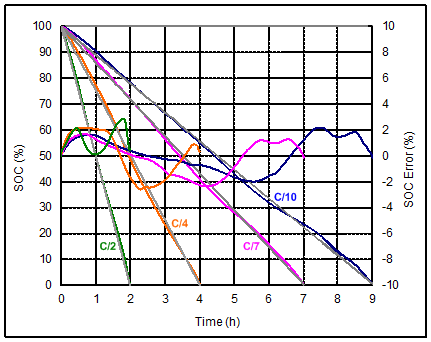Figure 13. Performance of the state of charge of the dynamic voltage algorithm under different discharge rates.

The figure below shows the performance of the state of charge when the battery is short-charged and short-discharged. The state of charge error is still very small, and the maximum error is only 3%.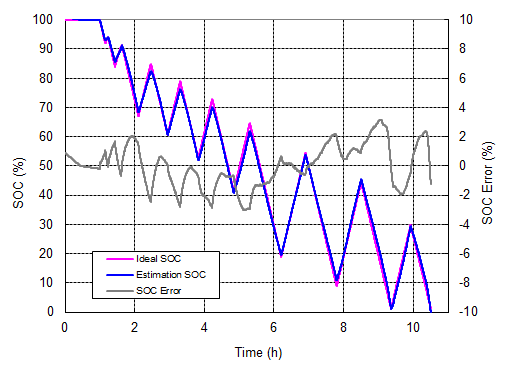Figure 14. In the case of short charge and short discharge of the battery, the performance of the state of charge of the dynamic voltage algorithm

Compared to the Coulomb counting method, which is prone to inaccuracies due to current sensing errors and battery self-discharge, the Dynamic Voltage Algorithm has a significant advantage in that it does not accumulate errors over time and current. This is a major benefit of the algorithm. However, since it lacks information about charge/discharge currents, the Dynamic Voltage Algorithm exhibits lower short-term accuracy and slower response time. Additionally, it is unable to estimate the full charge capacity. Nevertheless, it performs well in long-term accuracy because the battery voltage directly reflects its state of charge.